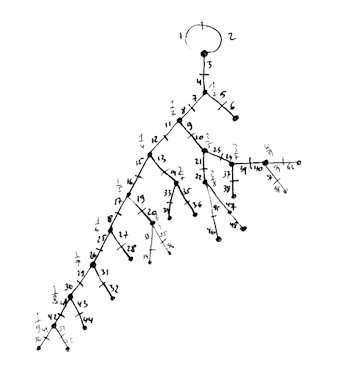Skip to content →

# Tag: hyperbolic

The Monster is the largest of the 26 sporadic simple groups and has order

808 017 424 794 512 875 886 459 904 961 710 757 005 754 368 000 000 000

= 2^46 3^20 5^9 7^6 11^2 13^3 17 19 23 29 31 41 47 59 71.

It is not so much the size of its order that makes it hard to do actual calculations in the monster, but rather the dimensions of its smallest non-trivial irreducible representations (196 883 for the smallest, 21 296 876 for the next one, and so on).

In characteristic two there is an irreducible representation of one dimension less (196 882) which appears to be of great use to obtain information. For example, Robert Wilson used it to prove that The Monster is a Hurwitz group. This means that the Monster is generated by two elements g and h satisfying the relations

$g^2 = h^3 = (gh)^7 = 1$

Geometrically, this implies that the Monster is the automorphism group of a Riemann surface of genus g satisfying the Hurwitz bound 84(g-1)=#Monster. That is,

g=9619255057077534236743570297163223297687552000000001=42151199 * 293998543 * 776222682603828537142813968452830193

Or, in analogy with the Klein quartic which can be constructed from 24 heptagons in the tiling of the hyperbolic plane, there is a finite region of the hyperbolic plane, tiled with heptagons, from which we can construct this monster curve by gluing the boundary is a specific way so that we get a Riemann surface with exactly 9619255057077534236743570297163223297687552000000001 holes. This finite part of the hyperbolic tiling (consisting of #Monster/7 heptagons) we’ll call the empire of the monster and we’d love to describe it in more detail.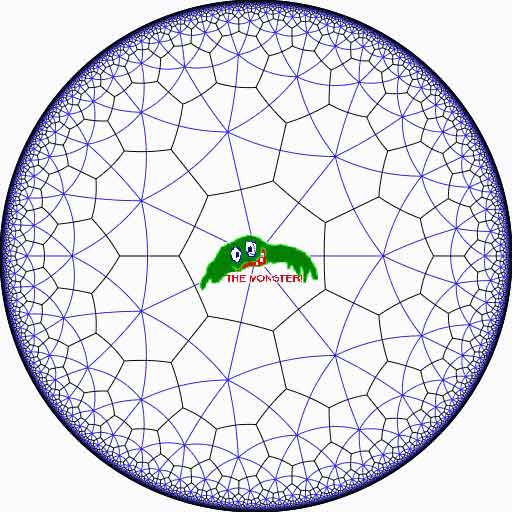Look at the half-edges of all the heptagons in the empire (the picture above learns that every edge in cut in two by a blue geodesic). They are exactly #Monster such half-edges and they form a dessin d’enfant for the monster-curve.

If we label these half-edges by the elements of the Monster, then multiplication by g in the monster interchanges the two half-edges making up a heptagonal edge in the empire and multiplication by h in the monster takes a half-edge to the one encountered first by going counter-clockwise in the vertex of the heptagonal tiling. Because g and h generated the Monster, the dessin of the empire is just a concrete realization of the monster.

Because g is of order two and h is of order three, the two permutations they determine on the dessin, gives a group epimorphism $C_2 \ast C_3 = PSL_2(\mathbb{Z}) \rightarrow \mathbb{M}$ from the modular group $PSL_2(\mathbb{Z})$ onto the Monster-group.

In noncommutative geometry, the group-algebra of the modular group $\mathbb{C} PSL_2$ can be interpreted as the coordinate ring of a noncommutative manifold (because it is formally smooth in the sense of Kontsevich-Rosenberg or Cuntz-Quillen) and the group-algebra of the Monster $\mathbb{C} \mathbb{M}$ itself corresponds in this picture to a finite collection of ‘points’ on the manifold. Using this geometric viewpoint we can now ask the question What does the Monster see of the modular group?

To make sense of this question, let us first consider the commutative equivalent : what does a point P see of a commutative variety X?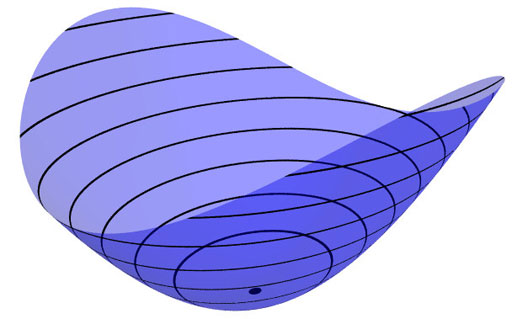Evaluation of polynomial functions in P gives us an algebra epimorphism $\mathbb{C}[X] \rightarrow \mathbb{C}$ from the coordinate ring of the variety $\mathbb{C}[X]$ onto $\mathbb{C}$ and the kernel of this map is the maximal ideal $\mathfrak{m}_P$ of
$\mathbb{C}[X]$ consisting of all functions vanishing in P.

Equivalently, we can view the point $P= \mathbf{spec}~\mathbb{C}[X]/\mathfrak{m}_P$ as the scheme corresponding to the quotient $\mathbb{C}[X]/\mathfrak{m}_P$. Call this the 0-th formal neighborhood of the point P.

This sounds pretty useless, but let us now consider higher-order formal neighborhoods. Call the affine scheme $\mathbf{spec}~\mathbb{C}[X]/\mathfrak{m}_P^{n+1}$ the n-th forml neighborhood of P, then the first neighborhood, that is with coordinate ring $\mathbb{C}[X]/\mathfrak{m}_P^2$ gives us tangent-information. Alternatively, it gives the best linear approximation of functions near P.
The second neighborhood $\mathbb{C}[X]/\mathfrak{m}_P^3$ gives us the best quadratic approximation of function near P, etc. etc.

These successive quotients by powers of the maximal ideal $\mathfrak{m}_P$ form a system of algebra epimorphisms

$\ldots \frac{\mathbb{C}[X]}{\mathfrak{m}_P^{n+1}} \rightarrow \frac{\mathbb{C}[X]}{\mathfrak{m}_P^{n}} \rightarrow \ldots \ldots \rightarrow \frac{\mathbb{C}[X]}{\mathfrak{m}_P^{2}} \rightarrow \frac{\mathbb{C}[X]}{\mathfrak{m}_P} = \mathbb{C}$

and its inverse limit $\underset{\leftarrow}{lim}~\frac{\mathbb{C}[X]}{\mathfrak{m}_P^{n}} = \hat{\mathcal{O}}_{X,P}$ is the completion of the local ring in P and contains all the infinitesimal information (to any order) of the variety X in a neighborhood of P. That is, this completion $\hat{\mathcal{O}}_{X,P}$ contains all information that P can see of the variety X.

In case P is a smooth point of X, then X is a manifold in a neighborhood of P and then this completion
$\hat{\mathcal{O}}_{X,P}$ is isomorphic to the algebra of formal power series $\mathbb{C}[[ x_1,x_2,\ldots,x_d ]]$ where the $x_i$ form a local system of coordinates for the manifold X near P.

Right, after this lengthy recollection, back to our question what does the monster see of the modular group? Well, we have an algebra epimorphism

$\pi~:~\mathbb{C} PSL_2(\mathbb{Z}) \rightarrow \mathbb{C} \mathbb{M}$

and in analogy with the commutative case, all information the Monster can gain from the modular group is contained in the $\mathfrak{m}$-adic completion

$\widehat{\mathbb{C} PSL_2(\mathbb{Z})}_{\mathfrak{m}} = \underset{\leftarrow}{lim}~\frac{\mathbb{C} PSL_2(\mathbb{Z})}{\mathfrak{m}^n}$

where $\mathfrak{m}$ is the kernel of the epimorphism $\pi$ sending the two free generators of the modular group $PSL_2(\mathbb{Z}) = C_2 \ast C_3$ to the permutations g and h determined by the dessin of the pentagonal tiling of the Monster’s empire.

As it is a hopeless task to determine the Monster-empire explicitly, it seems even more hopeless to determine the kernel $\mathfrak{m}$ let alone the completed algebra… But, (surprise) we can compute $\widehat{\mathbb{C} PSL_2(\mathbb{Z})}_{\mathfrak{m}}$ as explicitly as in the commutative case we have $\hat{\mathcal{O}}_{X,P} \simeq \mathbb{C}[[ x_1,x_2,\ldots,x_d ]]$ for a point P on a manifold X.

Here the details : the quotient $\mathfrak{m}/\mathfrak{m}^2$ has a natural structure of $\mathbb{C} \mathbb{M}$-bimodule. The group-algebra of the monster is a semi-simple algebra, that is, a direct sum of full matrix-algebras of sizes corresponding to the dimensions of the irreducible monster-representations. That is,

$\mathbb{C} \mathbb{M} \simeq \mathbb{C} \oplus M_{196883}(\mathbb{C}) \oplus M_{21296876}(\mathbb{C}) \oplus \ldots \ldots \oplus M_{258823477531055064045234375}(\mathbb{C})$

with exactly 194 components (the number of irreducible Monster-representations). For any $\mathbb{C} \mathbb{M}$-bimodule $M$ one can form the tensor-algebra

$T_{\mathbb{C} \mathbb{M}}(M) = \mathbb{C} \mathbb{M} \oplus M \oplus (M \otimes_{\mathbb{C} \mathbb{M}} M) \oplus (M \otimes_{\mathbb{C} \mathbb{M}} M \otimes_{\mathbb{C} \mathbb{M}} M) \oplus \ldots \ldots$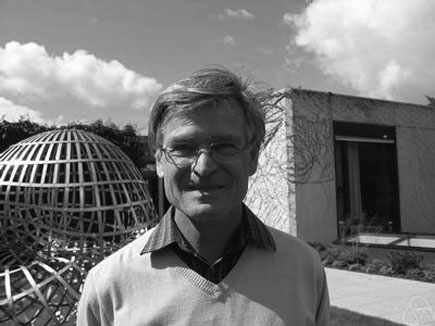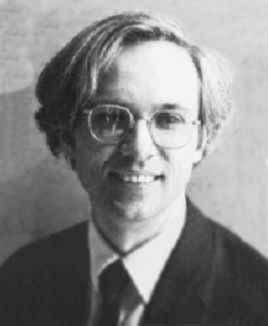and applying the formal neighborhood theorem for formally smooth algebras (such as $\mathbb{C} PSL_2(\mathbb{Z})$) due to Joachim Cuntz (left) and Daniel Quillen (right) we have an isomorphism of algebras

$\widehat{\mathbb{C} PSL_2(\mathbb{Z})}_{\mathfrak{m}} \simeq \widehat{T_{\mathbb{C} \mathbb{M}}(\mathfrak{m}/\mathfrak{m}^2)}$

where the right-hand side is the completion of the tensor-algebra (at the unique graded maximal ideal) of the $\mathbb{C} \mathbb{M}$-bimodule $\mathfrak{m}/\mathfrak{m}^2$, so we’d better describe this bimodule explicitly.

Okay, so what’s a bimodule over a semisimple algebra of the form $S=M_{n_1}(\mathbb{C}) \oplus \ldots \oplus M_{n_k}(\mathbb{C})$? Well, a simple S-bimodule must be either (1) a factor $M_{n_i}(\mathbb{C})$ with all other factors acting trivially or (2) the full space of rectangular matrices $M_{n_i \times n_j}(\mathbb{C})$ with the factor $M_{n_i}(\mathbb{C})$ acting on the left, $M_{n_j}(\mathbb{C})$ acting on the right and all other factors acting trivially.

That is, any S-bimodule can be represented by a quiver (that is a directed graph) on k vertices (the number of matrix components) with a loop in vertex i corresponding to each simple factor of type (1) and a directed arrow from i to j corresponding to every simple factor of type (2).

That is, for the Monster, the bimodule $\mathfrak{m}/\mathfrak{m}^2$ is represented by a quiver on 194 vertices and now we only have to determine how many loops and arrows there are at or between vertices.

Using Morita equivalences and standard representation theory of quivers it isn’t exactly rocket science to determine that the number of arrows between the vertices corresponding to the irreducible Monster-representations $S_i$ and $S_j$ is equal to

$dim_{\mathbb{C}}~Ext^1_{\mathbb{C} PSL_2(\mathbb{Z})}(S_i,S_j)-\delta_{ij}$

Now, I’ve been wasting a lot of time already here explaining what representations of the modular group have to do with quivers (see for example here or some other posts in the same series) and for quiver-representations we all know how to compute Ext-dimensions in terms of the Euler-form applied to the dimension vectors.

Right, so for every Monster-irreducible $S_i$ we have to determine the corresponding dimension-vector $~(a_1,a_2;b_1,b_2,b_3)$ for the quiver

$\xymatrix{ & & & & \vtx{b_1} \\ \vtx{a_1} \ar[rrrru]^(.3){B_{11}} \ar[rrrrd]^(.3){B_{21}} \ar[rrrrddd]_(.2){B_{31}} & & & & \\ & & & & \vtx{b_2} \\ \vtx{a_2} \ar[rrrruuu]_(.7){B_{12}} \ar[rrrru]_(.7){B_{22}} \ar[rrrrd]_(.7){B_{23}} & & & & \\ & & & & \vtx{b_3}}$

Now the dimensions $a_i$ are the dimensions of the +/-1 eigenspaces for the order 2 element g in the representation and the $b_i$ are the dimensions of the eigenspaces for the order 3 element h. So, we have to determine to which conjugacy classes g and h belong, and from Wilson’s paper mentioned above these are classes 2B and 3B in standard Atlas notation.

So, for each of the 194 irreducible Monster-representations we look up the character values at 2B and 3B (see below for the first batch of those) and these together with the dimensions determine the dimension vector $~(a_1,a_2;b_1,b_2,b_3)$.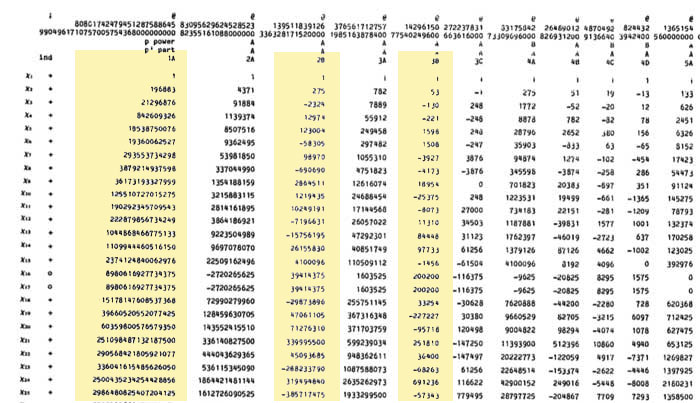For example take the 196883-dimensional irreducible. Its 2B-character is 275 and the 3B-character is 53. So we are looking for a dimension vector such that $a_1+a_2=196883, a_1-275=a_2$ and $b_1+b_2+b_3=196883, b_1-53=b_2=b_3$ giving us for that representation the dimension vector of the quiver above $~(98579,98304,65663,65610,65610)$.

Okay, so for each of the 194 irreducibles $S_i$ we have determined a dimension vector $~(a_1(i),a_2(i);b_1(i),b_2(i),b_3(i))$, then standard quiver-representation theory asserts that the number of loops in the vertex corresponding to $S_i$ is equal to

$dim(S_i)^2 + 1 – a_1(i)^2-a_2(i)^2-b_1(i)^2-b_2(i)^2-b_3(i)^2$

and that the number of arrows from vertex $S_i$ to vertex $S_j$ is equal to

$dim(S_i)dim(S_j) – a_1(i)a_1(j)-a_2(i)a_2(j)-b_1(i)b_1(j)-b_2(i)b_2(j)-b_3(i)b_3(j)$

This data then determines completely the $\mathbb{C} \mathbb{M}$-bimodule $\mathfrak{m}/\mathfrak{m}^2$ and hence the structure of the completion $\widehat{\mathbb{C} PSL_2}_{\mathfrak{m}}$ containing all information the Monster can gain from the modular group.

But then, one doesn’t have to go for the full regular representation of the Monster. Any faithful permutation representation will do, so we might as well go for the one of minimal dimension.

That one is known to correspond to the largest maximal subgroup of the Monster which is known to be a two-fold extension $2.\mathbb{B}$ of the Baby-Monster. The corresponding permutation representation is of dimension 97239461142009186000 and decomposes into Monster-irreducibles

$S_1 \oplus S_2 \oplus S_4 \oplus S_5 \oplus S_9 \oplus S_{14} \oplus S_{21} \oplus S_{34} \oplus S_{35}$

(in standard Atlas-ordering) and hence repeating the arguments above we get a quiver on just 9 vertices! The actual numbers of loops and arrows (I forgot to mention this, but the quivers obtained are actually symmetric) obtained were found after laborious computations mentioned in this post and the details I’ll make avalable here.

Anyone who can spot a relation between the numbers obtained and any other part of mathematics will obtain quantities of genuine (ie. non-Inbev) Belgian beer…

We are after the geometric trinity corresponding to the trinity of exceptional Galois groupsThe surfaces on the right have the corresponding group on the left as their group of automorphisms. But, there is a lot more group-theoretic info hidden in the geometry. Before we sketch the $L_2(11)$ case, let us recall the simpler situation of $L_2(7)$.

There are some excellent web-page on the Klein quartic and it would be too hard to try to improve on them, so we refer to John Baez’ page and Greg Egan’s page for more details.

The Klein quartic is the degree 4 projective plane curve defined by the equation $x^3y+y^3z+z^3x=0$. It can be tiled with a set of 24 regular heptagons, or alternatively with a set of 56 equilateral triangles and these two tilings are dual to each other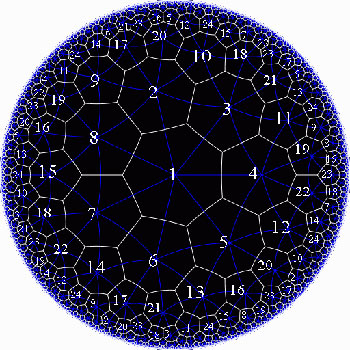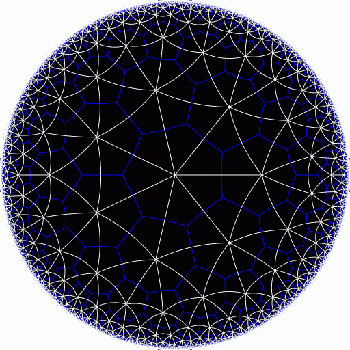In the triangular tiling, there are 56 triangles, 84 edges and 24 vertices. The 56 triangles come in 7 bunches of 8 each and we give the 7 bunches of triangles each a different color as in the pictures below made by Greg Egan. Observe that in the hyperbolic tiling all triangles look alike, but in the picture on the left most of them get warped as we try to embed the quartic in 3-space (which is impossible to do properly). The non-warped triangles (the red ones) come into pairs, the top and bottom triangles of a triangular prism, one prism at each of the four ‘vertices’ of a tetrahedron.

The automorphism group $L_2(7)$ acts on these triangles as $S_4$ acts on the triangles in a truncated cube.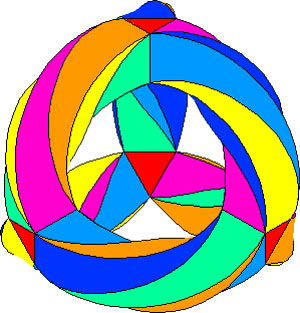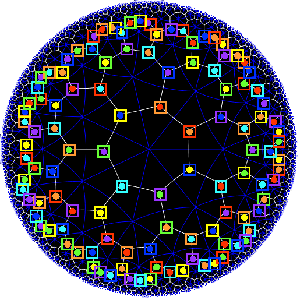The buckyball construction from a conjugacy class of order 11 elements from $L_2(11)$ recalled last time, has an analogon $L_2(7)$, leading to the truncated cube.

In $L_2(7)$ there are two conjugacy classes of subgroups isomorphic to $S_4$ (the rotation-symmetry group of the cube) as well as two conjugacy classes of order 7 elements, each consisting of precisely 24 elements, say C and D. The normalizer subgroup of C has order 21, so there is a cyclic group of order 3 acting non-trivially on the conjugacy class C with 8 orbits consisting of three elements each. These are the eight triangles of the truncated cube identified above as the red triangles.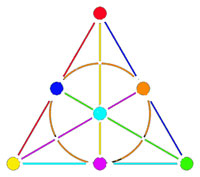Shifting perspective, we can repeat this for each of the seven different colors. That is, we have seven truncated cubes in the Klein quartic. On each of them a copy of $S_4$ acts and these subgroups form one of the two conjugacy classes of $S_4$ in the group $L_2(7)$. The colors of the triangles of these seven truncated cubes are indicated by bullets in the picture above on the right. The other conjugacy class of $S_4$’s act on ‘truncated anti-cubes’ which also come in seven bunches of which the color is indicated by a square in that picture.

If you spend enough time on it you will see that each (truncated) cube is completely disjoint from precisely 3 (truncated) anti-cubes. This reminds us of the Fano-plane (picture on the left) : it has 7 points (our seven truncated cubes), 7 lines (the truncated anti-cubes) and the incidence relation of points and lines corresponds to the disjointness of (truncated) cubes and anti-cubes! This is the geometric interpretation of the group-theoretic realization that $L_2(7) \simeq PGL_3(\mathbb{F}_2)$ is the isomorphism group of the projective plane over the finite field $\mathbb{F}_2$ on two elements, that is, the Fano plane. The colors of the picture on the left indicate the colors of cubes (points) and anti-cubes (lines) consistent with Egan’s picture above.

Further, the 24 vertices correspond to the 24 cusps of the modular group $\Gamma(7)$. Recall that a modular interpretation of the Klein quartic is as $\mathbb{H}/\Gamma(7)$ where $\mathbb{H}$ is the upper half-plane on which the modular group $\Gamma = PSL_2(\mathbb{Z})$ acts via Moebius transformations, that is, to a 2×2 matrix corresponds the transformation

$$\begin{bmatrix} a & b \\ c & d \end{bmatrix}$$ <----> $z \mapsto \frac{az+b}{cz+d}$

Okay, now let’s briefly sketch the exciting results found by Pablo Martin and David Singerman in the paper From biplanes to the Klein quartic and the buckyball, extending the above to the group $L_2(11)$.

There is one important modification to be made. Recall that the Cayley-graph to get the truncated cube comes from taking as generators of the group $S_4$ the set ${ (3,4),(1,2,3) }$, that is, an order two and an order three element, defining an epimorphism from the modular group $\Gamma= C_2 \ast C_3 \rightarrow S_4$.

We have also seen that in order to get the buckyball as a Cayley-graph for $A_5$ we need to take the generating set ${ (2,3)(4,5),(1,2,3,4,5) }$, so a degree two and a degree five element.

Hence, if we want to have a corresponding Riemann surface we’d better not start from the action of the modular group on the upper half-plane, but rather the action via Moebius transformations of the
Hecke group

$H^5 \simeq C_2 \ast C_5 = \langle z \mapsto -\frac{1}{z}, z \mapsto z+ \phi \rangle$

where $\phi = \frac{1 + \sqrt{5}}{2}$ is the golden ratio.

But then, there is an epimorphism $H^5 \rightarrow L_2(11)$ (as this group is generated by one element of degree 2 and one of degree 5) and let $\Lambda$ denote its kernel. Observe that $\Lambda$ is the analogon of the modular subgroup $\Gamma(7)$ used above to define the Klein quartic.

Hence, Martin and Singerman define the buckyball curve as the modular quotient $X=\mathbb{H}/\Lambda$ which is a Riemann surface of genus 70.

The terminlogy is motivated by the fact that, precisely as we got 7 truncated cubes in the Klein quartic, we now get 11 truncated icosahedra (that is, buckyballs) in $X$. The 11 coming, analogous to the Klein case, from thefact that there are precisely two conjugacy classes of subgroups of $L_2(11)$ isomorphic to $A_5$, each class containing precisely eleven elements!
The 60 vertices of the buckyball again correspond to the fact that there are 60 cusps in this case.

So, what is the analogon of the Fano plane in this case? Well, observe that the Fano-plane is a biplane of order two. That is, if we take as ‘points’ the points of the Fano plane and as ‘lines’ the complements of lines in the Fano plane then this defines a biplane structure. This means that any two distinct ‘points’ are contained in two distinct ‘lines’ and that two distinct ‘lines’ intersect in two distinct ‘points’. A biplane is said to be of order k is each ‘line’ consist of k-2 ‘points’. As the complement of a line in the Fano plane consists of 4 points, the Fano plane is therefore a biplane of order 2. The intersection pattern of cubes and anti-cubes in the Klein quartic is this biplane structure on the Fano plane.

In a similar way, Martin and Singerman show that the two conjugacy classes of subgroups isomorphic to $A_5$ in $L_2(11)$, each containing exactly 11 elements, correspond to 11 embedded buckyballs (and 11 anti-buckyballs) in the buckyball-curve $X$ and that the intersection relations among them describe the combinatorial structure of a biplane of order three if we view the 11 buckys as ‘points’ and the anti-buckys as ‘lines’.

That is, the buckyball curve is a perfect geometric counterpart of the Klein quartic for the two trinitiesAt the Arcadian Functor, Kea also has a post on this in which she conjectures that the Kac-Moody algebra of E11 may be related to the buckyball curve.

References :

David Singerman, “Klein’s Riemann surface of genus 3 and regular embeddings of finite projective planes” Bull. London Math. Soc. 18 (1986) 364-370.

Pablo Martin and David Singerman, “From biplanes to the Klein quartic and the Buckyball” (note that this is a preliminary version, please contact David Singerman for the latest version).Referring to the triple of exceptional Galois groups $L_2(5),L_2(7),L_2(11)$ and its connection to the Platonic solids I wrote : “It sure seems that surprises often come in triples…”. Briefly I considered replacing triples by trinities, but then, I didnt want to sound too mystic…

David Corfield of the n-category cafe and a dialogue on infinity (and perhaps other blogs I’m unaware of) pointed me to the paper Symplectization, complexification and mathematical trinities by Vladimir I. Arnold. (Update : here is a PDF-conversion of the paper)

The paper is a write-up of the second in a series of three lectures Arnold gave in june 1997 at the meeting in the Fields Institute dedicated to his 60th birthday. The goal of that lecture was to explain some mathematical dreams he had.

The next dream I want to present is an even more fantastic set of theorems and conjectures. Here I also have no theory and actually the ideas form a kind of religion rather than mathematics.
The key observation is that in mathematics one encounters many trinities. I shall present a list of examples. The main dream (or conjecture) is that all these trinities are united by some rectangular “commutative diagrams”.
I mean the existence of some “functorial” constructions connecting different trinities. The knowledge of the existence of these diagrams provides some new conjectures which might turn to be true theorems.

Follows a list of 12 trinities, many taken from Arnold’s field of expertise being differential geometry. I’ll restrict to the more algebraically inclined ones.

1 : “The first trinity everyone knows is”where $\mathbb{H}$ are the Hamiltonian quaternions. The trinity on the left may be natural to differential geometers who see real and complex and hyper-Kaehler manifolds as distinct but related beasts, but I’m willing to bet that most algebraists would settle for the trinity on the right where $\mathbb{O}$ are the octonions.

2 : The next trinity is that of the exceptional Lie algebras E6, E7 and E8.with corresponding Dynkin-Coxeter diagramsArnold has this to say about the apparent ubiquity of Dynkin diagrams in mathematics.

Manin told me once that the reason why we always encounter this list in many different mathematical classifications is its presence in the hardware of our brain (which is thus unable to discover a more complicated scheme).
I still hope there exists a better reason that once should be discovered.

Amen to that. I’m quite hopeful human evolution will overcome the limitations of Manin’s brain…

3 : Next comes the Platonic trinity of the tetrahedron, cube and dodecahedronClearly one can argue against this trinity as follows : a tetrahedron is a bunch of triangles such that there are exactly 3 of them meeting in each vertex, a cube is a bunch of squares, again 3 meeting in every vertex, a dodecahedron is a bunch of pentagons 3 meeting in every vertex… and we can continue the pattern. What should be a bunch a hexagons such that in each vertex exactly 3 of them meet? Well, only one possibility : it must be the hexagonal tiling (on the left below). And in normal Euclidian space we cannot have a bunch of septagons such that three of them meet in every vertex, but in hyperbolic geometry this is still possible and leads to the Klein quartic (on the right). Check out this wonderful post by John Baez for more on this.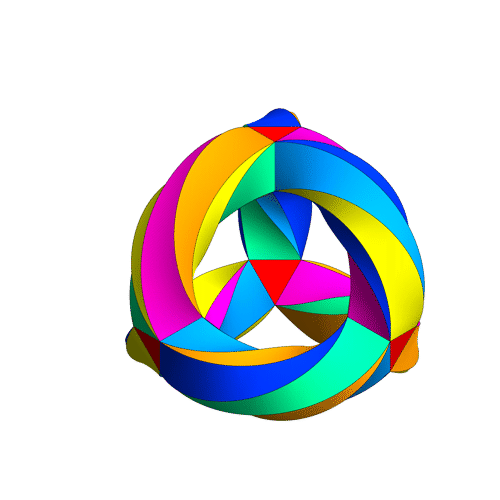4 : The trinity of the rotation symmetry groups of the three Platonicswhere $A_n$ is the alternating group on n letters and $S_n$ is the symmetric group.

Clearly, any rotation of a Platonic solid takes vertices to vertices, edges to edges and faces to faces. For the tetrahedron we can easily see the 4 of the group $A_4$, say the 4 vertices. But what is the 4 of $S_4$ in the case of a cube? Well, a cube has 4 body-diagonals and they are permuted under the rotational symmetries. The most difficult case is to see the $5$ of $A_5$ in the dodecahedron. Well, here’s the solution to this riddle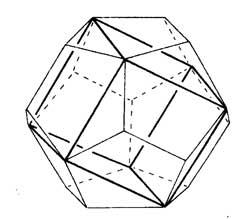there are exactly 5 inscribed cubes in a dodecahedron and they are permuted by the rotations in the same way as $A_5$.

7 : The seventh trinity involves complex polynomials in one variablethe Laurant polynomials and the modular polynomials (that is, rational functions with three poles at 0,1 and $\infty$.

8 : The eight one is another beautyHere ‘numbers’ are the ordinary complex numbers $\mathbb{C}$, the ‘trigonometric numbers’ are the quantum version of those (aka q-numbers) which is a one-parameter deformation and finally, the ‘elliptic numbers’ are a two-dimensional deformation. If you ever encountered a Sklyanin algebra this will sound familiar.

This trinity is based on a paper of Turaev and Frenkel and I must come back to it some time…

The paper has some other nice trinities (such as those among Whitney, Chern and Pontryagin classes) but as I cannot add anything sensible to it, let us include a few more algebraic trinities. The first one attributed by Arnold to John McKay

13 : A trinity parallel to the exceptional Lie algebra one isbetween the 27 straight lines on a cubic surface, the 28 bitangents on a quartic plane curve and the 120 tritangent planes of a canonic sextic curve of genus 4.

14 : The exceptional Galois groupsexplained last time.

15 : The associated curves with these groups as symmetry groups (as in the previous post)where the ? refers to the mysterious genus 70 curve. I’ll check with one of the authors whether there is still an embargo on the content of this paper and if not come back to it in full detail.

16 : The three generations of sporadic groupsDo you have other trinities you’d like to worship?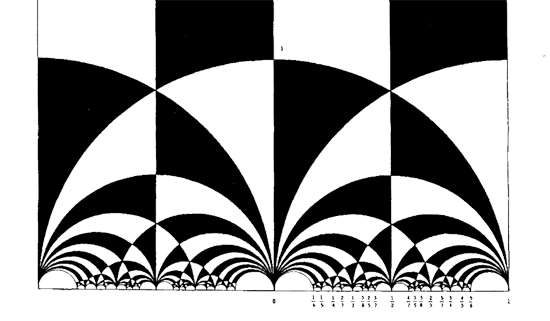The black&white psychedelic picture on the left of a tessellation of the hyperbolic upper-halfplane, was called the Dedekind tessellation in this post, following the reference given by John Stillwell in his excellent paper Modular Miracles, The American Mathematical Monthly, 108 (2001) 70-76.

But is this correct terminology? Nobody else uses it apparently. So, let’s try to track down the earliest depiction of this tessellation in the literature…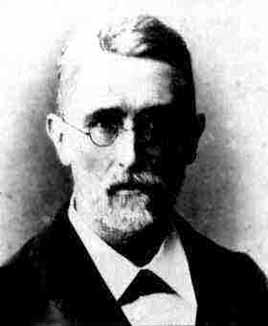Stillwell refers to Richard Dedekind‘s 1877 paper “Schreiben an Herrn Borchard uber die Theorie der elliptische Modulfunktionen”, which appeared beginning of september 1877 in Crelle’s journal (Journal fur die reine und angewandte Mathematik, Bd. 83, 265-292).

There are a few odd things about this paper. To start, it really is the transcript of a (lengthy) letter to Herrn Borchardt (at first, I misread the recipient as Herrn Borcherds which would be really weird…), written on June 12th 1877, just 2 and a half months before it appeared… Even today in the age of camera-ready-copy it would probably take longer.

There isn’t a single figure in the paper, but, it is almost impossible to follow Dedekind’s arguments without having a mental image of the tessellation. He gives a fundamental domain for the action of the modular group $\Gamma = PSL_2(\mathbb{Z})$ on the hyperbolic upper-half plane (a fact already known to Gauss) and goes on in section 3 to give a one-to-one mapping between this domain and the complex plane using what he calls the ‘valenz’ function $v$ (which is our modular function $j$, making an appearance in moonshine, and responsible for the black&white tessellation, the two colours corresponding to pre-images of the upper or lower half-planes).

Then there is this remarkable opening sentence.

Sie haben mich aufgefordert, eine etwas ausfuhrlichere Darstellung der Untersuchungen auszuarbeiten, von welchen ich, durch das Erscheinen der Abhandlung von Fuchs veranlasst, mir neulich erlaubt habe Ihnen eine kurze Ubersicht mitzuteilen; indem ich Ihrer Einladung hiermit Folge leiste, beschranke ich mich im wesentlichen auf den Teil dieser Untersuchungen, welcher mit der eben genannten Abhandlung zusammenhangt, und ich bitte Sie auch, die Ubergehung einiger Nebenpunkte entschuldigen zu wollen, da es mir im Augenblick an Zeit fehlt, alle Einzelheiten auszufuhren.

Well, just try to get a paper (let alone a letter) accepted by Crelle’s Journal with an opening line like : “I’ll restrict to just a few of the things I know, and even then, I cannot be bothered to fill in details as I don’t have the time to do so right now!” But somehow, Dedekind got away with it.

So, who was this guy Borchardt? How could this paper be published so swiftly? And, what might explain this extreme ‘je m’en fous’-opening ?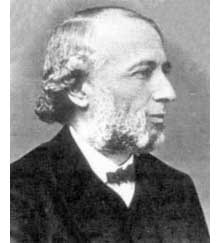Carl Borchardt was a Berlin mathematician whose main claim to fame seems to be that he succeeded Crelle in 1856 as main editor of the ‘Journal fur reine und…’ until 1880 (so in 1877 he was still in charge, explaining the swift publication). It seems that during this time the ‘Journal’ was often referred to as “Borchardt’s Journal” or in France as “Journal de M Borchardt”. After Borchardt’s death, the Journal für die Reine und Angewandte Mathematik again became known as Crelle’s Journal.

As to the opening sentence, I have a toy-theory of what was going on. In 1877 a bitter dispute was raging between Kronecker (an editor for the Journal and an important one as he was the one succeeding Borchardt when he died in 1880) and Cantor. Cantor had published most of his papers at Crelle and submitted his latest find : there is a one-to-one correspondence between points in the unit interval [0,1] and points of d-dimensional space! Kronecker did everything in his power to stop that paper to the extend that Cantor wanted to retract it and submit it elsewhere. Dedekind supported Cantor and convinced him not to retract the paper and used his influence to have the paper published in Crelle in 1878. Cantor greatly resented Kronecker’s opposition to his work and never submitted any further papers to Crelle’s Journal.

Clearly, Borchardt was involved in the dispute and it is plausible that he ‘invited’ Dedekind to submit a paper on his old results in the process. As a further peace offering, Dedekind included a few ‘nice’ words for Kronecker

Bei meiner Versuchen, tiefer in diese mir unentbehrliche Theorie einzudringen und mir einen einfachen Weg zu den ausgezeichnet schonen Resultaten von Kronecker zu bahnen, die leider noch immer so schwer zuganglich sind, enkannte ich sogleich…

Probably, Dedekind was referring to Kronecker’s relation between class groups of quadratic imaginary fields and the j-function, see the miracle of 163. As an added bonus, Dedekind was elected to the Berlin academy in 1880…

Anyhow, no visible sign of ‘Dedekind’s’ tessellation in the 1877 Dedekind paper, so, we have to look further. I’m fairly certain to have found the earliest depiction of the black&white tessellation (if you have better info, please drop a line). Here it is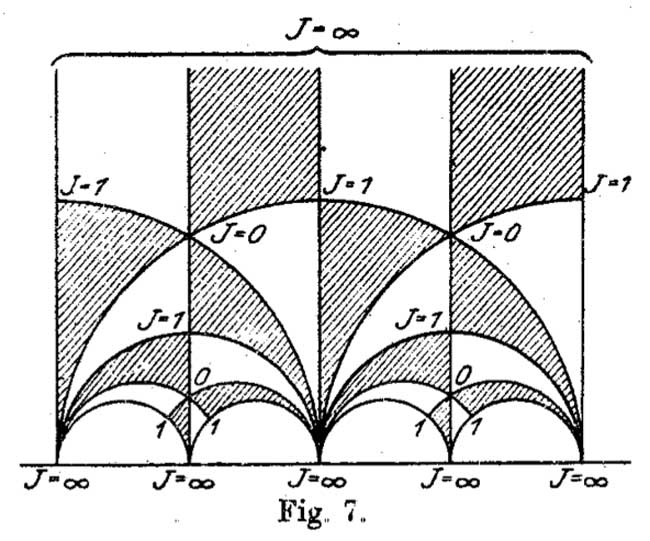It is figure 7 in Felix Klein‘s paper “Uber die Transformation der elliptischen Funktionen und die Auflosung der Gleichungen funften Grades” which appeared in may 1878 in the Mathematische Annalen (Bd. 14 1878/79). He even adds the j-values which make it clear why black triangles should be oriented counter-clockwise and white triangles clockwise. If Klein would still be around today, I’m certain he’d be a metapost-guru.

So, perhaps the tessellation should be called Klein’s tessellation??
Well, not quite. Here’s what Klein writes wrt. figure 7

Diese Figur nun – welche die eigentliche Grundlage fur das Nachfolgende abgibt – ist eben diejenige, von der Dedekind bei seiner Darstellung ausgeht. Er kommt zu ihr durch rein arithmetische Betrachtung.

Case closed : Klein clearly acknowledges that Dedekind did have this picture in mind when writing his 1877 paper!

But then, there are a few odd things about Klein’s paper too, and, I do have a toy-theory about this as well… (tbc)

It’s been a while, so let’s include a recap : a (transitive) permutation representation of the modular group $\Gamma = PSL_2(\mathbb{Z})$ is determined by the conjugacy class of a cofinite subgroup $\Lambda \subset \Gamma$, or equivalently, to a dessin d’enfant. We have introduced a quiver (aka an oriented graph) which comes from a triangulation of the compactification of $\mathbb{H} / \Lambda$ where $\mathbb{H}$ is the hyperbolic upper half-plane. This quiver is independent of the chosen embedding of the dessin in the Dedeking tessellation. (For more on these terms and constructions, please consult the series Modular subgroups and Dessins d’enfants).

Why are quivers useful? To start, any quiver $Q$ defines a noncommutative algebra, the path algebra $\mathbb{C} Q$, which has as a $\mathbb{C}$-basis all oriented paths in the quiver and multiplication is induced by concatenation of paths (when possible, or zero otherwise). Usually, it is quite hard to make actual computations in noncommutative algebras, but in the case of path algebras you can just see what happens.

Moreover, we can also see the finite dimensional representations of this algebra $\mathbb{C} Q$. Up to isomorphism they are all of the following form : at each vertex $v_i$ of the quiver one places a finite dimensional vectorspace $\mathbb{C}^{d_i}$ and any arrow in the quiver
$$\xymatrix{\vtx{v_i} \ar[r]^a & \vtx{v_j}}$$ determines a linear map between these vertex spaces, that is, to $a$ corresponds a matrix in $M_{d_j \times d_i}(\mathbb{C})$. These matrices determine how the paths of length one act on the representation, longer paths act via multiplcation of matrices along the oriented path.

A necklace in the quiver is a closed oriented path in the quiver up to cyclic permutation of the arrows making up the cycle. That is, we are free to choose the start (and end) point of the cycle. For example, in the one-cycle quiver

$$\xymatrix{\vtx{} \ar[rr]^a & & \vtx{} \ar[ld]^b \\ & \vtx{} \ar[lu]^c &}$$

the basic necklace can be represented as $abc$ or $bca$ or $cab$. How does a necklace act on a representation? Well, the matrix-multiplication of the matrices corresponding to the arrows gives a square matrix in each of the vertices in the cycle. Though the dimensions of this matrix may vary from vertex to vertex, what does not change (and hence is a property of the necklace rather than of the particular choice of cycle) is the trace of this matrix. That is, necklaces give complex-valued functions on representations of $\mathbb{C} Q$ and by a result of Artin and Procesi there are enough of them to distinguish isoclasses of (semi)simple representations! That is, linear combinations a necklaces (aka super-potentials) can be viewed, after taking traces, as complex-valued functions on all representations (similar to character-functions).

In physics, one views these functions as potentials and it then interested in the points (representations) where this function is extremal (minimal) : the vacua. Clearly, this does not make much sense in the complex-case but is relevant when we look at the real-case (where we look at skew-Hermitian matrices rather than all matrices). A motivating example (the Yang-Mills potential) is given in Example 2.3.2 of Victor Ginzburg’s paper Calabi-Yau algebras.

Let $\Phi$ be a super-potential (again, a linear combination of necklaces) then our commutative intuition tells us that extrema correspond to zeroes of all partial differentials $\frac{\partial \Phi}{\partial a}$ where $a$ runs over all coordinates (in our case, the arrows of the quiver). One can make sense of differentials of necklaces (and super-potentials) as follows : the partial differential with respect to an arrow $a$ occurring in a term of $\Phi$ is defined to be the path in the quiver one obtains by removing all 1-occurrences of $a$ in the necklaces (defining $\Phi$) and rearranging terms to get a maximal broken necklace (using the cyclic property of necklaces). An example, for the cyclic quiver above let us take as super-potential $abcabc$ (2 cyclic turns), then for example

$\frac{\partial \Phi}{\partial b} = cabca+cabca = 2 cabca$

(the first term corresponds to the first occurrence of $b$, the second to the second). Okay, but then the vacua-representations will be the representations of the quotient-algebra (which I like to call the vacualgebra)

$\mathcal{U}(Q,\Phi) = \frac{\mathbb{C} Q}{(\partial \Phi/\partial a, \forall a)}$

which in ‘physical relevant settings’ (whatever that means…) turn out to be Calabi-Yau algebras.

But, let us return to the case of subgroups of the modular group and their quivers. Do we have a natural super-potential in this case? Well yes, the quiver encoded a triangulation of the compactification of $\mathbb{H}/\Lambda$ and if we choose an orientation it turns out that all ‘black’ triangles (with respect to the Dedekind tessellation) have their arrow-sides defining a necklace, whereas for the ‘white’ triangles the reverse orientation makes the arrow-sides into a necklace. Hence, it makes sense to look at the cubic superpotential $\Phi$ being the sum over all triangle-sides-necklaces with a +1-coefficient for the black triangles and a -1-coefficient for the white ones. Let’s consider an index three example from a previous post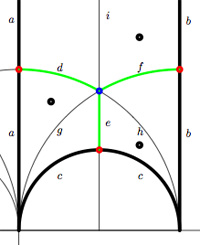$$\xymatrix{& & \rho \ar[lld]_d \ar[ld]^f \ar[rd]^e & \\ i \ar[rrd]_a & i+1 \ar[rd]^b & & \omega \ar[ld]^c \\ & & 0 \ar[uu]^h \ar@/^/[uu]^g \ar@/_/[uu]_i &}$$

In this case the super-potential coming from the triangulation is

$\Phi = -aid+agd-cge+che-bhf+bif$

and therefore we have a noncommutative algebra $\mathcal{U}(Q,\Phi)$ associated to this index 3 subgroup. Contrary to what I believed at the start of this series, the algebras one obtains in this way from dessins d’enfants are far from being Calabi-Yau (in whatever definition). For example, using a GAP-program written by Raf Bocklandt Ive checked that the growth rate of the above algebra is similar to that of $\mathbb{C}[x]$, so in this case $\mathcal{U}(Q,\Phi)$ can be viewed as a noncommutative curve (with singularities).

However, this is not the case for all such algebras. For example, the vacualgebra associated to the second index three subgroup (whose fundamental domain and quiver were depicted at the end of this post) has growth rate similar to that of $\mathbb{C} \langle x,y \rangle$…

I have an outlandish conjecture about the growth-behavior of all algebras $\mathcal{U}(Q,\Phi)$ coming from dessins d’enfants : the algebra sees what the monodromy representation of the dessin sees of the modular group (or of the third braid group).
I can make this more precise, but perhaps it is wiser to calculate one or two further examples…

Here I will go over the last post at a more leisurely pace, focussing on a couple of far more trivial examples. Here’s the goal : we want to assign a quiver-superpotential to any subgroup of finite index of the modular group. So fix such a subgroup $\Gamma’$ of the modular group $\Gamma=PSL_2(\mathbb{Z})$ and consider the associated permutation representation of $\Gamma$ on the left-cosets $\Gamma/\Gamma’$. As $\Gamma \simeq C_2 \ast C_3$ this representation is determined by the action of the order 2 and order 3 generators of the modular group. There are a number of combinatorial gadgets to control the subgroup $\Gamma’$ and the associated permutation representation : (generalized) Farey symbols and dessins d’enfants.

Recall that the modular group acts on the upper-halfplane (the ‘hyperbolic plane’) by Moebius transformations, so to any subgroup $\Gamma’$ we can associate a fundamental domain for its restricted action. The dessins and the Farey symbols give us a particular choice of these fundamental domains. Let us consider the two most trivial subgroups of all : the modular group itself (so $\Gamma/\Gamma$ is just one element and therefore the associated permutation representation is just the trivial representation) and the unique index two subgroup $\Gamma_2$ (so there are two cosets $\Gamma/\Gamma_2$ and the order 2 generator interchanges these two while the order 3 generator acts trivially on them). The fundamental domains of $\Gamma$ (left) and $\Gamma_2$ (right) are depicted below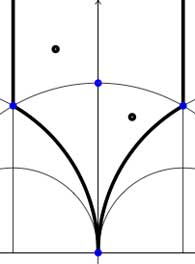In both cases the fundamental domain is bounded by the thick black (hyperbolic) edges. The left-domain consists of two hyperbolic triangles (the upper domain has $\infty$ as the third vertex) and the right-domain has 4 triangles. In general, if the subgroup $\Gamma’$ has index n, then its fundamental domain will consist of $2n$ hyperbolic triangles. Note that these triangles are part of the Dedekind tessellation so really depict the action of $PGL_2(\mathbb{Z}$ and any $\Gamma$-hyperbolic triangle consists of one black and one white triangle in Dedekind’s coloring. We will indicate the color of a triangle by a black circle if the corresponding triangle is black. Of course, the bounding edges of the fundamental domain need to be identified and the Farey symbol is a notation device to clarify this. The Farey symbols of the above domains are
$$\xymatrix{\infty \ar@{-}[r]_{\circ} & 0 \ar@{-}[r]_{\bullet} & \infty}$$ and $$\xymatrix{\infty \ar@{-}[r]_{\bullet} & 0 \ar@{-}[r]_{\bullet} & \infty}$$ respectively. In both cases this indicates that the two bounding edges on the left are to be identified as are the two bounding edges on the right (so, in particular, after identification $\infty$ coincides with $0$). Hence, after identification, the $\Gamma$ domain consists of two triangles on the vertices ${ 0,i,\rho }$ (where $\rho=e^{2 \pi i}{6}$) (the blue dots) sharing all three edges, the $\Gamma_2$ domain consists of 4 triangles on the 4 vertices ${ 0,i,\rho,\rho^2 }$ (the blue dots). In general we have three types of vertices : cusps (such as 0 or $\infty$), even vertices (such as $i$ where there are 4 hyperbolic edges in the Dedekind tessellation) and odd vertices (such as $\rho$ and $\rho^2$ where there are 6 hyperbolic edges in the tessellation).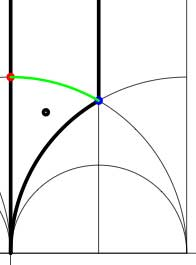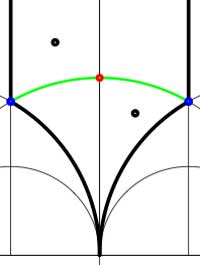Another combinatorial gadget assigned to the fundamental domain is the cuboid tree diagram or dessin. It consists of all odd and even vertices on the boundary of the domain, together with all odd and even vertices in the interior. These vertices are then connected with the hyperbolic edges connecting them. If we color the even vertices red and the odds blue we have the indicated dessins for our two examples (the green pictures). An half-edge is an edge connecting a red and a blue vertex in the dessin and we number all half-edges. So, the $\Gamma$-dessin has 1 half-edge whereas the $\Gamma_2$-dessin has two (in general, the number of these half-edges is equal to the index of the subgroup). Observe also that every triangle has exactly one half-edge as one of its three edges. The dessin gives all information to calculate the permutation representation on the coset-set $\Gamma/\Gamma’$ : the action of the order 2 generator of $\Gamma$ is given by taking for each internal red vertex the two-cycle $~(a,b)$ where a and b are the numbers of the two half-edges connected to the red vertex and the action of the order 3 generator is given by taking for every internal blue vertex the three cycle $~(c,d,e)$ where c, d and e are the numbers of the three half-edges connected to the blue vertex in counter-clockwise ordering. Our two examples above are a bit too simplistic to view this in action. There are no internal blue vertices, so the action of the order 3 generator is trivial in both cases. For $\Gamma$ there is also no red internal vertex, whence this is indeed the trivial representation whereas for $\Gamma_2$ there is one internal red vertex, so the action of the order 2 generator is given by $~(1,2)$, which is indeed the representation representation on $\Gamma/\Gamma_2$. In general, if the index of the subgroup $\Gamma’$ is n, then we call the subgroup of the symmetric group on n letters $S_n$ generated by the action-elements of the order 2 and order 3 generator the monodromy group of the permutation representation (or of the subgroup). In the trivial cases here, the monodromy groups are the trivial group (for $\Gamma$) and $C_2$ (for $\Gamma_2$).

As a safety-check let us work out all these concepts in the next simplest examples, those of some subgroups of index 3. Consider the Farey symbols

$$\xymatrix{\infty \ar@{-}[r]_{\circ} & 0 \ar@{-}[r]_{\circ} & 1 \ar@{-}[r]_{\circ} & \infty}$$ and
$$\xymatrix{\infty \ar@{-}[r]_{\circ} & 0 \ar@{-}[r]_{1} & 1 \ar@{-}[r]_{1} & \infty}$$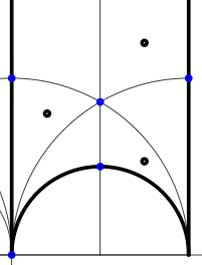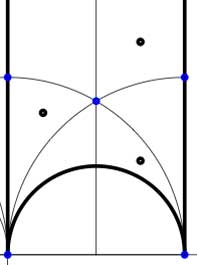In these cases the fundamental domain consists of 6 triangles with the indicated vertices (the blue dots). The distinction between the two is that in the first case, one identifies the two edges of the left, resp. bottom, resp. right boundary (so, in particular, 0,1 and $\infty$ are identified) whereas in the second one identifies the two edges of the left boundary and identifies the edges of the bottom with those of the right boundary (here, 0 is identified only with $\infty$ but also $1+i$ is indetified with $\frac{1}{2}+\frac{1}{2}i$).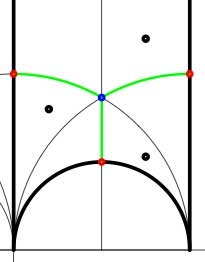In both cases the dessin seems to be the same (and given by the picture on the right). However, in the first case all three red vertices are distinct hence there are no internal red vertices in this case whereas in the second case we should identify the bottom and right-hand red vertex which then becomes an internal red vertex of the dessin!

Hence, if we order the three green half-edges 1,2,3 starting with the bottom one and counting counter-clockwise we see that in both cases the action of the order 3-generator of $\Gamma$ is given by the 3-cycle $~(1,2,3)$. The action of the order 2-generator is trivial in the first case, while given by the 2-cycle $~(1,2)$ in the second case. Therefore, the monodromy group is the cylic group $C_3$ in the first case and is the symmetric group $S_3$ in the second case.

Next time we will associate a quiver to these vertices and triangles as well as a cubic superpotential which will then allow us to define a noncommutative algebra associated to any subgroup of the modular group. The monodromy group of the situation will then reappear as a group of algebra-automorphisms of this noncommutative algebra!

Yesterday, Jan Stienstra gave a talk at theARTS entitled “Quivers, superpotentials and Dimer Models”. He started off by telling that the talk was based on a paper he put on the arXiv Hypergeometric Systems in two Variables, Quivers, Dimers and Dessins d’Enfants but that he was not going to say a thing about dessins but would rather focuss on the connection with superpotentials instead…pleasing some members of the public, while driving others to utter despair.

Anyway, it gave me the opportunity to figure out for myself what dessins might have to do with dimers, whathever these beasts are. Soon enough he put on a slide containing the definition of a dimer and from that moment on I was lost in my own thoughts… realizing that a dessin d’enfant had to be a dimer for the Dedekind tessellation of its associated Riemann surface!
and a few minutes later I could slap myself on the head for not having thought of this before :

There is a natural way to associate to a Farey symbol (aka a permutation representation of the modular group) a quiver and a superpotential (aka a necklace) defining (conjecturally) a Calabi-Yau algebra! Moreover, different embeddings of the cuboid tree diagrams in the hyperbolic plane may (again conjecturally) give rise to all sorts of arty-farty fanshi-wanshi dualities…

I’ll give here the details of the simplest example I worked out during the talk and will come back to general procedure later, when I’ve done a reference check. I don’t claim any originality here and probably all of this is contained in Stienstra’s paper or in some physics-paper, so if you know of a reference, please leave a comment. Okay, remember the Dedekind tessellation ?So, all hyperbolic triangles we will encounter below are colored black or white. Now, take a Farey symbol and consider its associated special polygon in the hyperbolic plane. If we start with the Farey symbol

$$\xymatrix{\infty \ar@{-}_{(1)}[r] & 0 \ar@{-}_{\bullet}[r] & 1 \ar@{-}_{(1)}[r] & \infty}$$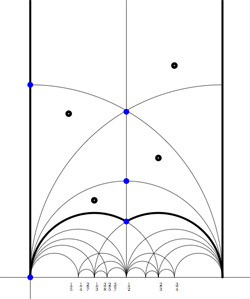we get the special polygonal region bounded by the thick edges, the vertical edges are identified as are the two bottom edges. Hence, this fundamental domain has 6 vertices (the 5 blue dots and the point at $i \infty$) and 8 hyperbolic triangles (4 colored black, indicated by a black dot, and 4 white ones).

Right, now let us associate a quiver to this triangulation (which embeds the quiver in the corresponding Riemann surface). The vertices of the triangulation are also the vertices of the quiver (so in our case we are going for a quiver with 6 vertices). Every hyperbolic edge in the triangulation gives one arrow in the quiver between the corresponding vertices. The orientation of the arrow is determined by the color of a triangle of which it is an edge : if the triangle is black, we run around its edges counter-clockwise and if the triangle is white we run over its edges clockwise (that is, the orientation of the arrow is independent of the choice of triangles to determine it). In our example, there is one arrows directed from the vertex at $i$ to the vertex at $0$, whether you use the black triangle on the left to determine the orientation or the white triangle on the right. If we do this for all edges in the triangulation we arrive at the quiver below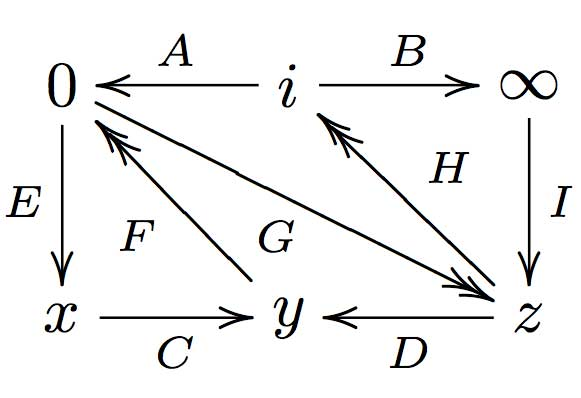where x,y and z are the three finite vertices on the $\frac{1}{2}$-axis from bottom to top and where I’ve used the physics-convention for double arrows, that is there are two F-arrows, two G-arrows and two H-arrows. Observe that the quiver is of Calabi-Yau type meaning that there are as much arrows coming into a vertex as there are arrows leaving the vertex.

Now that we have our quiver we determine the superpotential as follows. Fix an orientation on the Riemann surface (for example counter-clockwise) and sum over all black triangles the product of the edge-arrows counterclockwise MINUS sum over all white triangles
the product of the edge arrows counterclockwise. So, in our example we have the cubic superpotential

$IH’B+HAG+G’DF+FEC-BHI-H’G’A-GFD-CEF’$

From this we get the associated noncommutative algebra, which is the quotient of the path algebra of the above quiver modulo the following ‘commutativity relations’

$\begin{cases} GH &=G’H’ \\ IH’ &= IH \\ FE &= F’E \\ F’G’ &= FG \\ CF &= CF’ \\ EC &= GD \\ G’D &= EC \\ HA &= DF \\ DF’ &= H’A \\ AG &= BI \\ BI &= AG’ \end{cases}$

and morally this should be a Calabi-Yau algebra (( can someone who knows more about CYs verify this? )). This concludes the walk through of the procedure. Summarizing : to every Farey-symbol one associates a Calabi-Yau quiver and superpotential, possibly giving a Calabi-Yau algebra!

Here the details of the iguanodon series. Start with the Farey sequence $F(n)$of order n which is the sequence of completely reduced fractions between 0 and 1 which, when in lowest terms, have denominators less than or equal to n, arranged in order of increasing size. Here are the first eight Fareys

F(1) = {0⁄1, 1⁄1}
F(2) = {0⁄1, 1⁄2, 1⁄1}
F(3) = {0⁄1, 1⁄3, 1⁄2, 2⁄3, 1⁄1}
F(4) = {0⁄1, 1⁄4, 1⁄3, 1⁄2, 2⁄3, 3⁄4, 1⁄1}
F(5) = {0⁄1, 1⁄5, 1⁄4, 1⁄3, 2⁄5, 1⁄2, 3⁄5, 2⁄3, 3⁄4, 4⁄5, 1⁄1}
F(6) = {0⁄1, 1⁄6, 1⁄5, 1⁄4, 1⁄3, 2⁄5, 1⁄2, 3⁄5, 2⁄3, 3⁄4, 4⁄5, 5⁄6, 1⁄1}
F(7) = {0⁄1, 1⁄7, 1⁄6, 1⁄5, 1⁄4, 2⁄7, 1⁄3, 2⁄5, 3⁄7, 1⁄2, 4⁄7, 3⁄5, 2⁄3, 5⁄7, 3⁄4, 4⁄5, 5⁄6, 6⁄7, 1⁄1}
F(8) = {0⁄1, 1⁄8, 1⁄7, 1⁄6, 1⁄5, 1⁄4, 2⁄7, 1⁄3, 3⁄8, 2⁄5, 3⁄7, 1⁄2, 4⁄7, 3⁄5, 5⁄8, 2⁄3, 5⁄7, 3⁄4, 4⁄5, 5⁄6, 6⁄7, 7⁄8, 1⁄1}Farey sequences have plenty of mysterious properties. For example, in 1924 J. Franel and Edmund Landau proved that an asymptotic density result about Farey sequences is equivalent to the Riemann hypothesis.
More precisely, let a(n) be the number of terms in the Farey sequence F(n) (that is, a(1)=2,a(2)=3,…,a(8)=23 etc. This is sequence A005728 in the online integer sequences catalog).
Let $F(n)_j$ denote the j-th term in F(n), then the following conjecture is equivalent to the Riemann hypothesis

For every $\epsilon > 0$ there is a constant C depending on $\epsilon$ such that

$\sum_{j=1}^{a(n)} | F(n)_j – \frac{j}{a(n)} | < C n^{\frac{1}{2}+\epsilon}$

when n goes to infinity. Anyway, let us continue our construction. Farey sequences are clearly symmetric around 1/2 so let us just take half of them, so we jump to 1 when we have reached 1/2. Let us extend this halved Farey on both sides with $\infty$ and call it the modified Farey sequence f(n). For example,

$f(3) = {~\infty,0,\frac{1}{3},\frac{1}{2},1,\infty }$

Now consider the Farey code in which we identify the two sides connected to $\infty$ and mark two consecutive Farey numbers as

$$\xymatrix{f(n)_i \ar@{-}[r]_{\bullet} & f(n)_{i+1}}$$

That is, the Farey code associated to the modified sequence f(3) is

$$\xymatrix{\infty \ar@{-}[r]_{1} & 0 \ar@{-}[r]_{\bullet} & \frac{1}{3} \ar@{-}[r]_{\bullet} & \frac{1}{2} \ar@{-}[r]_{\bullet} & 1 \ar@{-}[r]_{1} & \infty}$$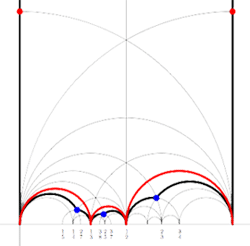Recall from earlier that to a Farey-code we can associate a special polygon by first taking the hyperbolic convex hull of all the terms in the sequence (the region bounded by the vertical lines and the bottom red circles in the picture on the left) and adding to it for each odd interval $$\xymatrix{f(n)_i \ar@{-}[r]_{\bullet} & f(n)_{i+1}}$$ the triangle just outside the convex hull consisting of two odd edges in the Dedekind tessellation (then we obtain the region bounded by the black geodesics for the sequence f(3)).

Next, we can associate to this special polygon a cuboid tree diagram by considering all even and odd vertices on the boundary (which are tinted red, respectively blue) together with all odd vertices in the interior of the special polygon. These are indicated in the left picture below. If we connect these vertices with the geodesics in the polygon we get a cuboid tree diagram. The obtained cuboid tree diagram is depicted on the right below.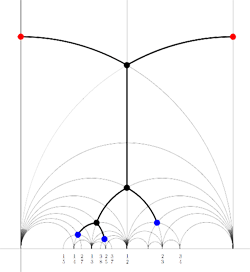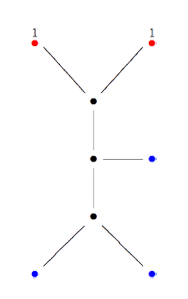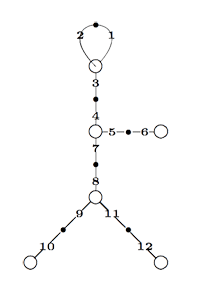Finally, identifying the red points (as they lie on geodesics connected to $\infty$ which are identified in the Farey code), adding even points on the remaining geodesics and numbering the obtained half-lines we obtain the dessin d’enfant given on the left hand side. To such a dessin we can associate its monodromy group which is a permutation group on the half-lines generated by an order two element indicating which half-lines make up a line and an order three element indicating which half-lines one encounters by walking counter-clockwise around a three-valent vertex. For the dessin on the left the group is therefore the subgroup of $S_{12}$ generated by the elements

$\alpha = (1,2)(3,4)(5,6)(7,8)(9,10)(11,12)$

$\beta = (1,2,3)(4,5,7)(8,9,11)$

and a verification with GAP tells us that this group is the sporadic Mathieu group $M_{12}$. This concludes the description of the second member of the Iguanodon series. If you like to check that the first 8 iguanodons are indeed the simple groups

$L_2(7), M_{12}, A_{16}, M_{24}, A_{28}, A_{40}, A_{48}, A_{60}, \ldots$

the following dissection of the Iguanodon may prove useful